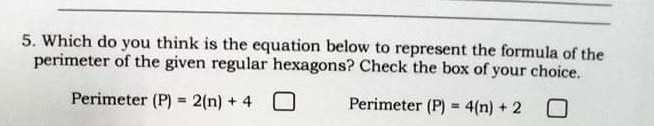### ¿Todavía tienes preguntas de matemáticas?

Pregunte a nuestros tutores expertos
Algebra
Pregunta5. Which do you think is the equation below to represent the formula of the perimeter of the given regular hexagons? Check the box of your choice.

Perimeter $$( P ) = 2 ( n ) + 4$$

Perimeter $$( P ) = 4 ( n ) + 2$$